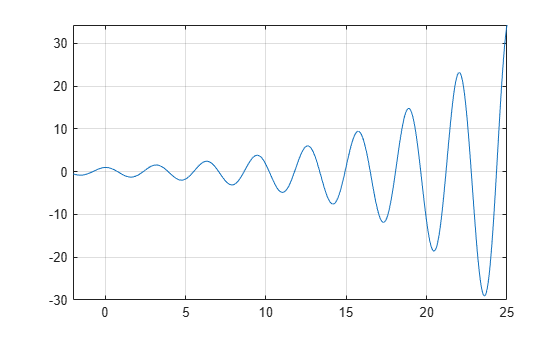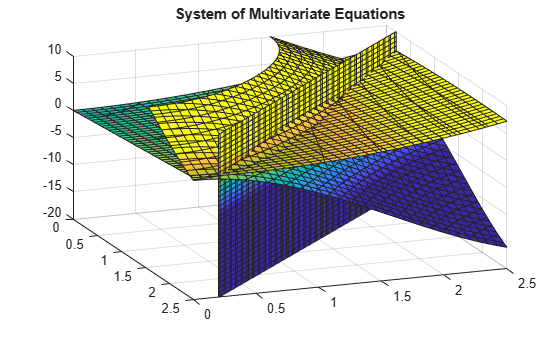# Solve Equations Numerically

Symbolic Math Toolbox™ offers both numeric and symbolic equation solvers. For a comparison of numeric and symbolic solvers, see Select Numeric or Symbolic Solver. An equation or a system of equations can have multiple solutions. To find these solutions numerically, use the function `vpasolve`. For polynomial equations, `vpasolve` returns all solutions. For nonpolynomial equations, `vpasolve` returns the first solution it finds. These examples show you how to use `vpasolve` to find solutions to both polynomial and nonpolynomial equations, and how to obtain these solutions to arbitrary precision.

### Find All Roots of a Polynomial Function

Use `vpasolve` to find all the solutions to the function $f\left(x\right)=6{x}^{7}-2{x}^{6}+3{x}^{3}-8$.

```syms f(x) f(x) = 6*x^7-2*x^6+3*x^3-8; sol = vpasolve(f)```
```sol =  $\left(\begin{array}{c}1.0240240759053702941448316563337\\ -0.88080620051762149639205672298326+0.50434058840127584376331806592405 \mathrm{i}\\ -0.88080620051762149639205672298326-0.50434058840127584376331806592405 \mathrm{i}\\ -0.22974795226118163963098570610724+0.96774615576744031073999010695171 \mathrm{i}\\ -0.22974795226118163963098570610724-0.96774615576744031073999010695171 \mathrm{i}\\ 0.7652087814927846556172932675903+0.83187331431049713218367239317121 \mathrm{i}\\ 0.7652087814927846556172932675903-0.83187331431049713218367239317121 \mathrm{i}\end{array}\right)$```

`vpasolve` returns seven roots of the function, as expected, because the function is a polynomial of degree seven.

### Find Zeros of a Nonpolynomial Function Using Search Ranges and Starting Points

A plot of the function $f\left(x\right)={e}^{\left(x/7\right)}\mathrm{cos}\left(2x\right)$ reveals periodic zeros, with increasing slopes at the zero points as $x$ increases.

```syms x h = fplot(exp(x/7)*cos(2*x),[-2 25]); grid on```Use `vpasolve` to find a zero of the function `f`. Note that `vpasolve` returns only one solution of a nonpolynomial equation, even if multiple solutions exist. On repeated calls, `vpasolve` returns the same result.

```f = exp(x/7)*cos(2*x); for k = 1:3 vpasolve(f,x) end```
`ans = $-7.0685834705770347865409476123789$`
`ans = $-7.0685834705770347865409476123789$`
`ans = $-7.0685834705770347865409476123789$`

To find multiple solutions, set the option `'Random'` to `true`. This makes `vpasolve` choose starting points randomly. For information on the algorithm that chooses random starting points, see Algorithms on the `vpasolve` page.

```for k = 1:3 vpasolve(f,x,'Random',true) end```
`ans = $-226.98006922186256147892598444194$`
`ans = $98.174770424681038701957605727484$`
`ans = $52.621676947629036744249276669932$`

To find a zero close to $x=10$, set the starting point to `10`.

`vpasolve(f,x,10)`
`ans = $10.210176124166828025003590995658$`

To find a zero close to $x=1000$, set the starting point to `1000`.

`vpasolve(f,x,1000)`
`ans = $999.8118620049516981407362567287$`

To find a zero in the range $15\le x\le 25$, set the search range to `[15 25]`.

`vpasolve(f,x,[15 25])`
`ans = $21.205750411731104359622842837137$`

To find multiple zeros in the range `[15 25]`, you cannot call `vpasolve` repeatedly because it returns the same result on each call, as previously shown. Instead, set the search range and set `'Random'` to `true`.

```for k = 1:3 vpasolve(f,x,[15 25],'Random',true) end```
`ans = $21.205750411731104359622842837137$`
`ans = $21.205750411731104359622842837137$`
`ans = $16.493361431346414501928877762217$`

Because `'Random'` selects starting points randomly, the same solution might be found on successive calls.

#### Find All Zeros in a Specified Search Range

Create a function `findzeros` to systematically find all zeros for `f` in a given search range, within a specified error tolerance. The function starts with the input search range and calls `vpasolve` to find a zero. Then, it splits the search range into two around the zero value and recursively calls itself with the new search ranges as inputs to find more zeros.

The function is explained section by section here.

Declare the function with the three inputs and one output. The first input is the function, the second input is the range, and the optional third input allows you to specify the error between a zero and the higher and lower bounds generated from it.

```function sol = findzeros(f,range,err) ```

If you do not specify the optional argument for error tolerance, `findzeros` sets `err` to `0.001`.

```if nargin < 2 err = 1e-3; end ```

Find a zero in the search range using `vpasolve`.

```sol = vpasolve(f,range); ```

If `vpasolve` does not find a zero, exit.

```if(isempty(sol)) return ```

If `vpasolve` finds a zero, split the search range into two search ranges above and below the zero.

```else lowLimit = sol-err; highLimit = sol+err; ```

Call `findzeros` with the lower search range. If `findzeros` returns zeros, copy the values into the solution array and sort them.

``` temp = findzeros(f,[range(1) lowLimit],1); if ~isempty(temp) sol = sort([sol temp]); end ```

Call `findzeros` with the higher search range. If `findzeros` returns zeros, copy the values into the solution array and sort them.

``` temp = findzeros(f,[highLimit range(2)],1); if ~isempty(temp) sol = sort([sol temp]); end return end end ```

The entire function `findzeros` is as follows. Save this function as `findzeros.m` in the current folder.

```function sol = findzeros(f,range,err) if nargin < 3 err = 1e-3; end sol = vpasolve(f,range); if(isempty(sol)) return else lowLimit = sol-err; highLimit = sol+err; temp = findzeros(f,[range(1) lowLimit],1); if ~isempty(temp) sol = sort([sol temp]); end temp = findzeros(f,[highLimit range(2)],1); if ~isempty(temp) sol = sort([sol temp]); end return end end ```

Call `findzeros` with search range `[15 25]` to find all zeros in that range for `f(x) = exp(x/7)*cos(2*x)`, within the default error tolerance.

```syms f(x) f(x) = exp(x/7)*cos(2*x); sol = findzeros(f,[15 25])'```
```sol =  $\left(\begin{array}{c}16.493361431346414501928877762217\\ 18.064157758141311121160199453857\\ 19.634954084936207740391521145497\\ 21.205750411731104359622842837137\\ 22.776546738526000978854164528776\\ 24.347343065320897598085486220416\end{array}\right)$```

### Obtain Solutions to Arbitrary Precision

Use `digits` to set the precision of the solutions returned by `vpasolve`. By default, `vpasolve` returns solutions to a precision of 32 significant figures.

```f = exp(x/7)*cos(2*x); vpasolve(f)```
`ans = $-7.0685834705770347865409476123789$`

Use `digits` to increase the precision to 64 significant figures. When modifying `digits`, ensure that you save its current value so that you can restore it.

```digitsOld = digits; digits(64) vpasolve(f)```
`ans = $-7.068583470577034786540947612378881489443631148593988097193625333$`

Next, change the precision of the solutions to 16 significant figures.

`digits(16)`

### Solve Multivariate Equations Using Search Ranges

Consider the following system of equations.

`$\begin{array}{l}\mathit{z}=10\left(\mathrm{cos}\left(\mathit{x}\right)+\mathrm{cos}\left(\mathit{y}\right)\right)\\ \mathit{z}=\mathit{x}+{\mathit{y}}^{2}-0.1{\mathit{x}}^{2}\mathit{y}\\ \mathit{x}+\mathit{y}-2.7=0\end{array}$`

A plot of the equations for $0\le x\le 2.5$ and $0\le x\le 2.5$ shows that the three surfaces intersect in two points. To better visualize the plot, use `view`. To scale the colormap values, use `caxis`.

```syms x y z eqn1 = z == 10*(cos(x) + cos(y)); eqn2 = z == x+y^2-0.1*x^2*y; eqn3 = x+y-2.7 == 0; equations = [eqn1 eqn2 eqn3]; fimplicit3(equations) axis([0 2.5 0 2.5 -20 10]) title('System of Multivariate Equations') view(69, 28) caxis([-15 10])```Use `vpasolve` to find a point where the surfaces intersect. The function `vpasolve` returns a structure. To access the `x`-, `y`-, and `z`-values of the solution, index into the structure.

```sol = vpasolve(equations); [sol.x sol.y sol.z]```
`ans = $\left(\begin{array}{ccc}2.369747722454798& 0.3302522775452021& 2.293354376823228\end{array}\right)$`

To search a region of the solution space, specify search ranges for the variables. If you specify the ranges $0\le x\le 1.5$ and $1.5\le y\le 2.5$, then `vpasolve` function searches the bounded area shown.Use `vpasolve` to find a solution for this search range. To omit a search range for $z$, set the third search range to `[NaN NaN]`.

```vars = [x y z]; range = [0 1.5; 1.5 2.5; NaN NaN]; sol = vpasolve(equations, vars, range); [sol.x sol.y sol.z]```
`ans = $\left(\begin{array}{ccc}0.9106266172563336& 1.789373382743666& 3.964101572135625\end{array}\right)$`

To find multiple solutions, set the `'Random'` option to `true`. This makes `vpasolve` use random starting points on successive runs. The `'Random'` option can be used in conjunction with search ranges to make `vpasolve` use random starting points within a search range. Because `'Random'` selects starting points randomly, the same solution might be found on successive calls. Call `vpasolve` repeatedly to ensure you find both solutions.

```clear sol range = [0 3; 0 3; NaN NaN]; for k = 1:5 temp = vpasolve(equations,vars,range,'Random',true); sol(k,1) = temp.x; sol(k,2) = temp.y; sol(k,3) = temp.z; end sol```
```sol =  $\left(\begin{array}{ccc}2.369747722454798& 0.3302522775452021& 2.293354376823228\\ 2.369747722454798& 0.3302522775452021& 2.293354376823228\\ 2.369747722454798& 0.330252277545202& 2.293354376823228\\ 0.9106266172563336& 1.789373382743666& 3.964101572135625\\ 0.9106266172563336& 1.789373382743666& 3.964101572135625\end{array}\right)$```

Plot the equations. Superimpose the solutions as a scatter plot of points with yellow `X` markers using `scatter3`. To better visualize the plot, make two of the surfaces transparent using `alpha`. Scale the colormap to the plot values using `caxis`, and change the perspective using `view`.

`vpasolve` finds solutions at the intersection of the surfaces formed by the equations as shown.

```clf ax = axes; h = fimplicit3(equations); h(2).FaceAlpha = 0; h(3).FaceAlpha = 0; axis([0 2.5 0 2.5 -20 10]) hold on scatter3(sol(:,1),sol(:,2),sol(:,3),600,'yellow','X','LineWidth',2) title('Randomly Found Solutions in Specified Search Range') cz = ax.Children; caxis([0 20]) view(69,28) hold off```Lastly, restore the old value of `digits` for further calculations.

`digits(digitsOld)`